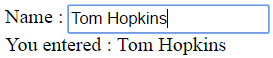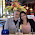Support us .Net Basics C# SQL ASP.NET ADO.NET MVC Slides C# Programs Subscribe Buy DVD

### Two way data binding in angular 2

Suggested Videos
Part 12 - Class binding in angular 2 | Text | Slides

In this video we will discuss Two way Data Binding in Angular 2.

Consider the following code in app.component.ts

import { Component } from '@angular/core';

@Component({
selector: 'my-app',
template: `
Name : <input [value]='name'>
<br>
You entered : {{name}}
`
})
export class AppComponent {
name: string = 'Tom';
}

• <input [value]='name'> : Binds component class "name" property to the input element’s value property
• You entered : {{name}} : Interpolation displays the value we have in "name" property on the web page

Here is the outputAt the moment when we change the value in the textbox, that changed value is not reflected in the browser. One way to achieve this is by binding to the input event of the input control as shown below.

Name : <input [value]='name' (input)='name = \$event.target.value'>
<br>
You entered : {{name}}

At this point, as we type in the textbox, the changed value is displayed on the page.So let's understand what is happening here. Conside this code

Name : <input [value]='name' (input)='name = \$event.target.value'>
<br>
You entered : {{name}}
• [value]='name' : This property binding flows data from the component class to element property
• (input)='name = \$event.target.value' : This event binding flows data in the opposite direction i.e from the element to component class property "name"
• \$event - Is exposed by angular event binding, and contains the event data. To retrieve the value from the input element use - \$event.target.value.
• name = \$event.target.value - This expression updates the value in the name property in the component class
• You entered : {{name}} - This interpolation expression will then display the value on the web page.
So in short two-way data binding in Angular is a combination of both Property Binding and Event Binding. To save a few keystrokes and simplify two-way data binding angular has provided ngModel directive. So with ngModel directive we can rewrite the following line of code
Name : <input [value]='name' (input)='name = \$event.target.value'>

Like this : Name : <input [(ngModel)]='name'>

At this point if you view the page in the browser, you will get the following error
Template parse errors:
Can't bind to 'ngModel' since it isn't a known property of 'input'

This is because ngModel directive is, in an Angular system module called FormsModule. For us to be able to use ngModel directive in our root module - AppModule, we will have to import FormsModule first.

Here are the steps to import FormsModule into our AppModule
1. Open app.module.ts file
2. Include the following import statement in it
import { FormsModule } from '@angular/forms';
3. Also, include FormsModule in the 'imports' array of @NgModule
imports: [BrowserModule, FormsModule]

With these changes, reload the web page and it will work as expected.

So here is the syntax for using two-way data binding in Angular
<input [(ngModel)]='name'>
• The square brackets on the outside are for property binding
• The parentheses on the inside are for event binding
• To easily remember this syntax, compare it to a banana in a box [()]1.2.